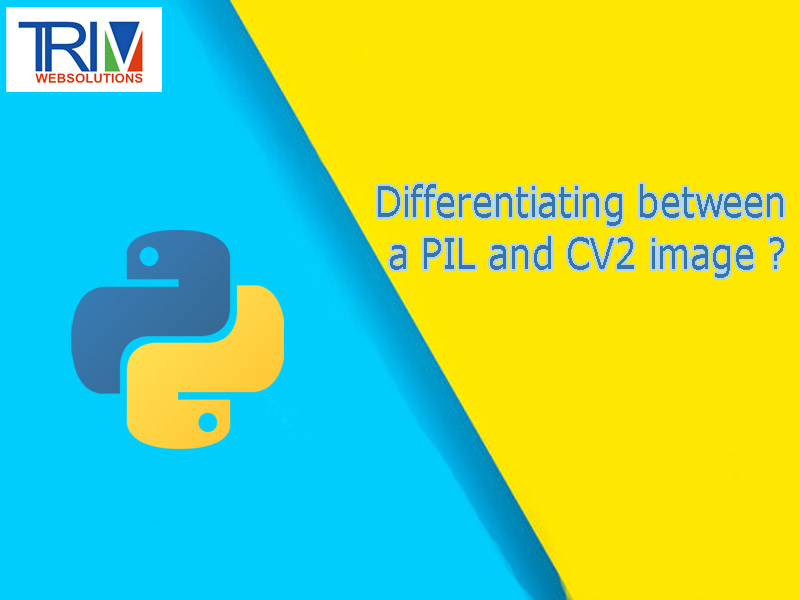Quick Inquiry

# Differentiating between a PIL and CV2 image in python ?When you open an image using `CV2` then it returns object of the type `numpy.ndarray` but PIL's `Image.open()` returns `PIL.JpegImagePlugin.JpegImageFile`. So using that, you can basically differentiate which is used in your case (assuming there is no conversion or further processing involved which converted one image type to another).

``` ```import cv2
from PIL import Image
from PIL import JpegImagePlugin

print(type(imgcv))

imgpil = Image.open('./koala.jpg')
print(type(imgpil))

img = imgpil
if isinstance(img,JpegImagePlugin.JpegImageFile):
print('PIL Image')
else:
print('Not PIL') ``````

Output:

``` ```<class 'numpy.ndarray'>
<class 'PIL.JpegImagePlugin.JpegImageFile'>
PIL Image ``````

As mentioned in the comment of this answer (by Mark Setchell) that instead of changing the PIL's `JpegImageFile` class, we can also check `ndarray` check and then decide - since PIL's class might change in future or they may simply use different type. The check would be exactly the same though.

``` ```img = imgcv
if isinstance(img,numpy.ndarray):
print('CV Image')
else:
print('PIL Image')``````